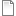Software:
Tag:
Video Duration:
5 minutes
Author:
Zach Downey

This is the first in a series of advanced scripting tutorials.  Before taking this one on, make sure you check out the intro tutorials first.  This is a really short video that just describes how the component operates.  I'll spare you all the pain of whatching me type out all the code.  Instead, check out the code below, or download the .gh file.

```//Set the branch angle by converting it from degs to radians
double bAngRad = ((Math.PI / 180) * branchAngle);
List<Line> lines = new List<Line>();
//Create a new line in the yDirection from the origin and add it to the list
Point3d stPt = new Point3d(0, 0, 0);
Vector3d unitYvect = new Vector3d(0, 1, 0);
Line ln0 = new Line(stPt, unitYvect, length);
//Create two temporary lists and set the first equal to the lines list
List<Line> tempList = new List<Line>();
List<Line> tempList2 = new List<Line>();
tempList = lines;

int i = 0;
while(i < num){
tempList = tempList2;
i++;
}
A = lines;```
```//<Custom additional code>

public List<Line> CreateBranch(List<Line> lines, double bScale, double bAng)
{
List<Line> newLines = new List<Line>();
foreach(Line ln in lines){
//Get the length of the current trunk and create a new scaled branch
double newLength = ln.Length * bScale;
//Get the endPt of the trunk and it's tangent vector
Point3d endPt = ln.To;
Vector3d unitTan = ln.UnitTangent;
//Create two new lines and make the second line have a negative rotation angle
Line ln1 = new Line(endPt, unitTan, newLength);
ln1.Transform(Rhino.Geometry.Transform.Rotation(bAng, endPt));
Line ln2 = new Line(endPt, unitTan, newLength);
ln2.Transform(Rhino.Geometry.Transform.Rotation(bAng * -1, endPt));
//Add these new branches to the list
}
return newLines;
}```
Tutorial Files:branching.gh

### Rating

Please rate this tutorial below. Thanks.